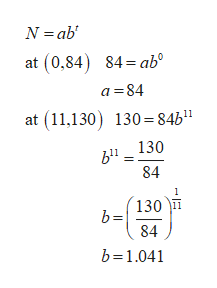# The number of asthma sufferers in the world was about 84 million in 1990 and 130 million in 2001. Let N represent the number of asthma sufferers (in millions) worldwide t years after 1990.part a. Write N as a linear function of t. Use exact values in your formula, not decimal approximations.part b. Write N as an exponential function of t. Use exact values in your formula, not decimal approximationspart c. How many asthma sufferers are predicted worldwide in the year 2018 with the linear model?

Question
31 views

The number of asthma sufferers in the world was about 84 million in 1990 and 130 million in 2001. Let N represent the number of asthma sufferers (in millions) worldwide t years after 1990.

part a. Write N as a linear function of t. Use exact values in your formula, not decimal approximations.

part b. Write N as an exponential function of t. Use exact values in your formula, not decimal approximations

part c. How many asthma sufferers are predicted worldwide in the year 2018 with the linear model?

check_circle

Step 1

(a) It is given that the asthma patients were 84 million in 1990 and 130 million in 2001.Let N represent number of asthma patient after 1990.Let the coordinate points be (0,84) and (11,130).Obtain N as a linear function of t.

Step 2

Use the slope and the point (0,84) to find the linear function of t.

Step 3

(b) Use the points (0,84) and (11,130) to obta...help_outlineImage TranscriptioncloseN ab at (0,84) 84 ab° a 84 at (11,130 130 84b1" b1130 84 130 84 b 1.041 fullscreen

### Want to see the full answer?

See Solution

#### Want to see this answer and more?

Solutions are written by subject experts who are available 24/7. Questions are typically answered within 1 hour.*

See Solution
*Response times may vary by subject and question.
Tagged in

### Other